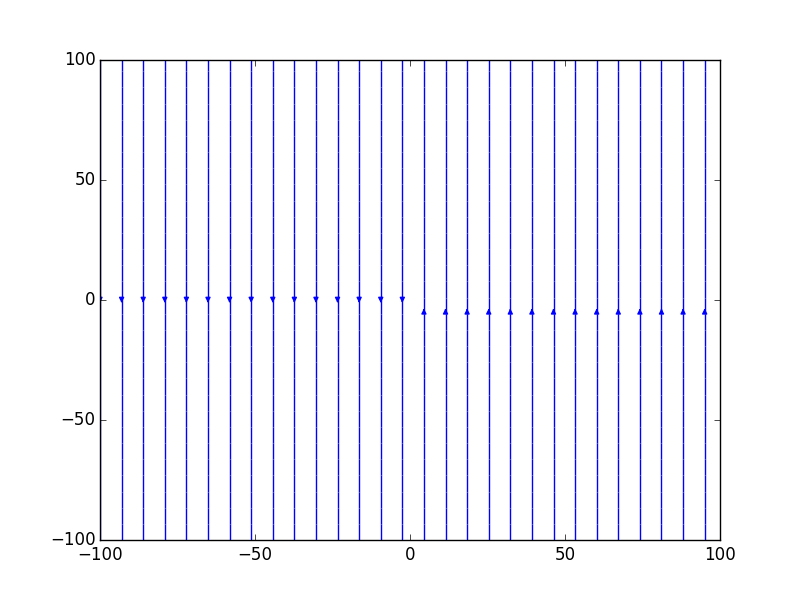# streamplot: vectors not pointing in the right direction!

Hi,

I have problems with streamplot

I want to use a 3d vector field in coordinates (x,y,z) stored in a numpy array, and plot slices of it with streamplot.

To test it I wanted to use a vector field with arrows pointed up in the z>0 region and pointed down in the z<0 region.

``````import numpy as np

import matplotlib.pyplot as plt

from math import *

max = 100

min = -100

X = np.linspace(min, max, num=100)

Y = np.linspace(min, max, num=100)

Z = np.linspace(min, max, num=100)

N = X.size

#single components in the 3D matrix

Bxa = np.zeros((N, N, N))

Bya = np.zeros((N, N, N))

Bza = np.zeros((N, N, N))

for i, x in enumerate(X):

for j, y in enumerate(Y):

for k, z in enumerate(Z):

Bxa[ i, j, k] = 0.0 #x

Bya[ i, j, k] = 0.0 #y

Bza[ i, j, k] = z

#I take a slice close to Y=0

Bx_sec = Bxa[:,N/2,:]

By_sec = Bya[:,N/2,:]

Bz_sec = Bza[:,N/2,:]

fig = plt.figure()

ax.streamplot(X, Z, Bx_sec, Bz_sec, color='b')

ax.set_xlim([X.min(), X.max()])

ax.set_ylim([Z.min(), Z.max()])

plt.show()
``````

But I obtain something that looks like if I have put Bza = x! I tried to invert the order of vectors but it is unuseful!

I attach the picture. Do you understand why? (the code I posted should run)

GabrieleThe documentation for streamplot:

``````        *x*, *y* : 1d arrays
an *evenly spaced* grid.
*u*, *v* : 2d arrays
x and y-velocities. Number of rows should match length of y, and
the number of columns should match x.
``````

Note that the rows in u and v should match y, and the columns should match x. I think your u and v are transposed.

Cheers!

Ben Root

···

On Fri, May 22, 2015 at 2:50 AM, Gabriele Brambilla <gb.gabrielebrambilla@…2015…87…> wrote:

Hi,

I have problems with streamplot

I want to use a 3d vector field in coordinates (x,y,z) stored in a numpy array, and plot slices of it with streamplot.

To test it I wanted to use a vector field with arrows pointed up in the z>0 region and pointed down in the z<0 region.

``````import numpy as np
``````
``````import matplotlib.pyplot as plt
``````
``````from math import *
``````
``````max = 100
``````
``````min = -100
``````
``````X = np.linspace(min, max, num=100)
``````
``````Y = np.linspace(min, max, num=100)
``````
``````Z = np.linspace(min, max, num=100)
``````
``````N = X.size
``````
``````#single components in the 3D matrix
``````
``````Bxa = np.zeros((N, N, N))
``````
``````Bya = np.zeros((N, N, N))
``````
``````Bza = np.zeros((N, N, N))
``````
``````for i, x in enumerate(X):
``````
``````	for j, y in enumerate(Y):
``````
``````		for k, z in enumerate(Z):
``````
``````			Bxa[ i, j, k] = 0.0 #x
``````
``````			Bya[ i, j, k] = 0.0 #y
``````
``````			Bza[ i, j, k] = z
``````
``````#I take a slice close to Y=0
``````
``````Bx_sec = Bxa[:,N/2,:]
``````
``````By_sec = Bya[:,N/2,:]
``````
``````Bz_sec = Bza[:,N/2,:]
``````
``````fig = plt.figure()
``````
``````ax = fig.add_subplot(111)
``````
``````ax.streamplot(X, Z, Bx_sec, Bz_sec, color='b')
``````
``````ax.set_xlim([X.min(), X.max()])
``````
``````ax.set_ylim([Z.min(), Z.max()])
``````
``````plt.show()
``````

But I obtain something that looks like if I have put Bza = x! I tried to invert the order of vectors but it is unuseful!

I attach the picture. Do you understand why? (the code I posted should run)

Gabriele

One dashboard for servers and applications across Physical-Virtual-Cloud

Widest out-of-the-box monitoring support with 50+ applications

Performance metrics, stats and reports that give you Actionable Insights

Deep dive visibility with transaction tracing using APM Insight.

Matplotlib-users mailing list

Matplotlib-users@lists.sourceforge.net

https://lists.sourceforge.net/lists/listinfo/matplotlib-users

Thanks! you are right!
I didn’t notice this detail

Gabriele

···

On Fri, May 22, 2015 at 3:58 PM, Benjamin Root <ben.root@…1304…> wrote:

The documentation for streamplot:

``````        *x*, *y* : 1d arrays
an *evenly spaced* grid.
*u*, *v* : 2d arrays
x and y-velocities. Number of rows should match length of y, and
the number of columns should match x.
``````

Note that the rows in u and v should match y, and the columns should match x. I think your u and v are transposed.

Cheers!

Ben Root

On Fri, May 22, 2015 at 2:50 AM, Gabriele Brambilla <gb.gabrielebrambilla@…287…> wrote:

Hi,

I have problems with streamplot

I want to use a 3d vector field in coordinates (x,y,z) stored in a numpy array, and plot slices of it with streamplot.

To test it I wanted to use a vector field with arrows pointed up in the z>0 region and pointed down in the z<0 region.

``````import numpy as np
``````
``````import matplotlib.pyplot as plt
``````
``````from math import *
``````
``````max = 100
``````
``````min = -100
``````
``````X = np.linspace(min, max, num=100)
``````
``````Y = np.linspace(min, max, num=100)
``````
``````Z = np.linspace(min, max, num=100)
``````
``````N = X.size
``````
``````#single components in the 3D matrix
``````
``````Bxa = np.zeros((N, N, N))
``````
``````Bya = np.zeros((N, N, N))
``````
``````Bza = np.zeros((N, N, N))
``````
``````for i, x in enumerate(X):
``````
``````	for j, y in enumerate(Y):
``````
``````		for k, z in enumerate(Z):
``````
``````			Bxa[ i, j, k] = 0.0 #x
``````
``````			Bya[ i, j, k] = 0.0 #y
``````
``````			Bza[ i, j, k] = z
``````
``````#I take a slice close to Y=0
``````
``````Bx_sec = Bxa[:,N/2,:]
``````
``````By_sec = Bya[:,N/2,:]
``````
``````Bz_sec = Bza[:,N/2,:]
``````
``````fig = plt.figure()
``````
``````ax = fig.add_subplot(111)
``````
``````ax.streamplot(X, Z, Bx_sec, Bz_sec, color='b')
``````
``````ax.set_xlim([X.min(), X.max()])
``````
``````ax.set_ylim([Z.min(), Z.max()])
``````
``````plt.show()
``````

But I obtain something that looks like if I have put Bza = x! I tried to invert the order of vectors but it is unuseful!

I attach the picture. Do you understand why? (the code I posted should run)

Gabriele

One dashboard for servers and applications across Physical-Virtual-Cloud

Widest out-of-the-box monitoring support with 50+ applications

Performance metrics, stats and reports that give you Actionable Insights

Deep dive visibility with transaction tracing using APM Insight.